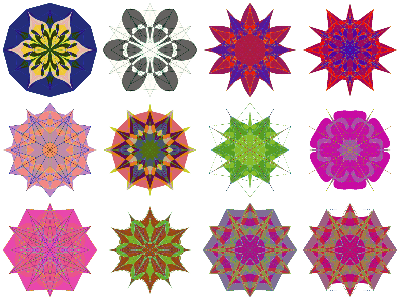#Function Repository Resource:

# RandomMandala

Make random mandala plots

Contributed by: Anton Antonov
 ResourceFunction["RandomMandala"][] generates a mandala graphic.

## Details and Options

The mandalas made by ResourceFunction["RandomMandala"] are generated through rotational symmetry of a “seed segment”.
RandomMandala accepts the same options as Graphics as well as the following options to specify different features of the seed segment generation and rotational symmetry order:
 "RotationalSymmetryOrder" 6 a value k defining the rotation symmetry angle, 2 π/k "Radius" 10 maximum radius of the seed segment generation points "SymmetricSeed" should the seed be symmetric or not "NumberOfSeedElements" Automatic the number of elements in a seed segment "KeepGridPoints" should the grid points be shown "ConnectionFunction" Random how to connect grid point "ColorFunction" how to colorize the mandala
The option "ConnectionFunction" specifies what Graphics primitive function should be used to connect the grid points of the seed segment. If the value is Automatic then Polygon is used. If the value is Random then a random choice is made from the following: Line, Polygon, BezierCurve, FilledCurve[BezierCurve[#]]&.

## Examples

### Basic Examples

Here we generate a random mandala:

 In:=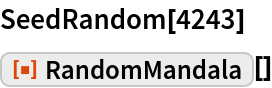Out=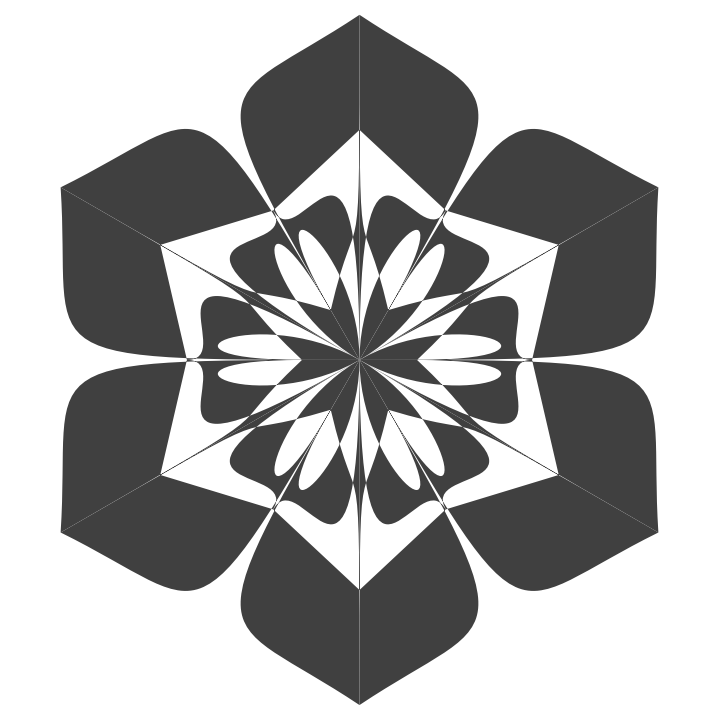Here we generate a mandala with different option settings:

 In:=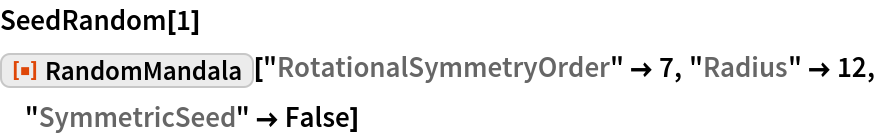Out=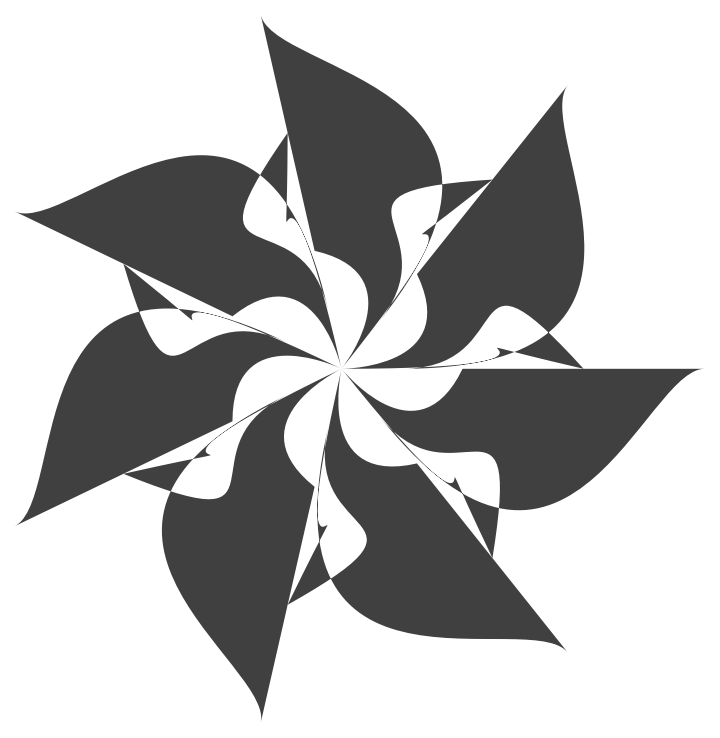### Scope

There are two modes of making random mandalas: (i) single-mandala mode and (ii) multi-mandala mode. The multi-mandala mode is activated by giving the “Radius” option a list of positive reals.

Here are gray-level and colorized mandalas generated with the single-mandala mode:

 In:=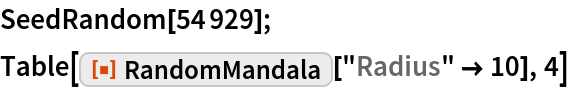Out=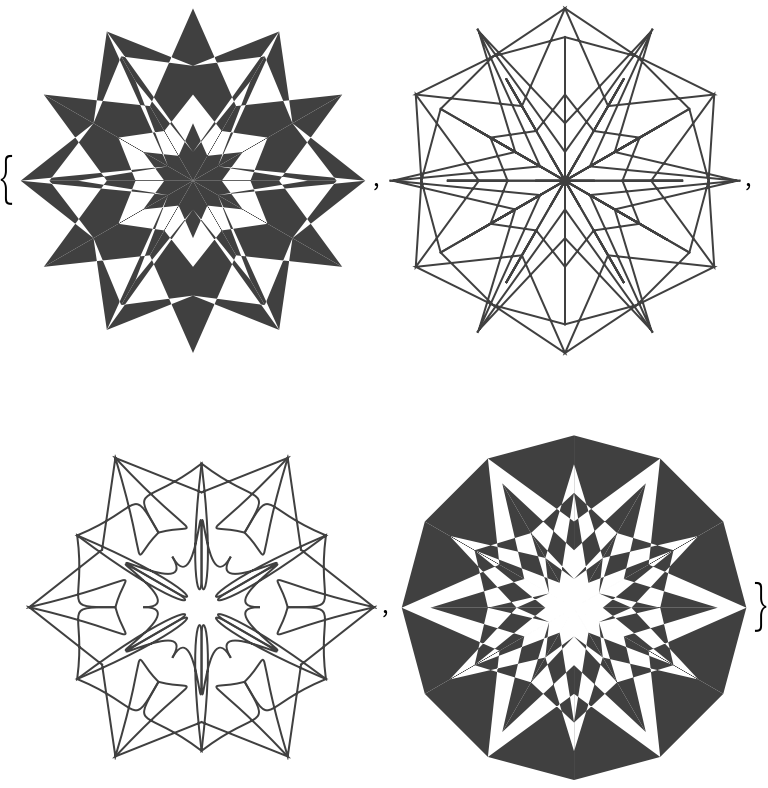In:=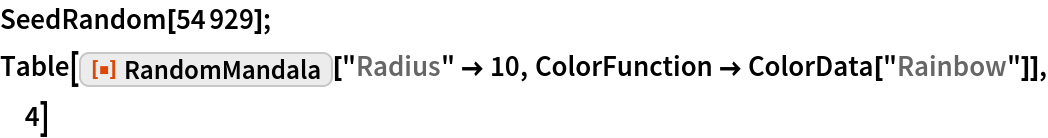Out=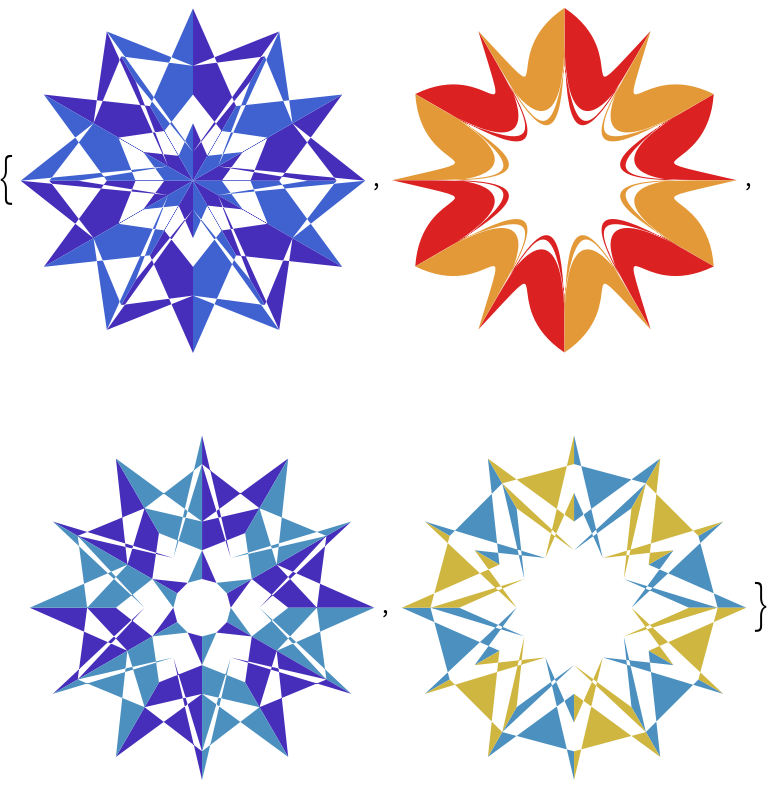Here are gray-level and colorized mandalas generated with a multi-mandala mode:

 In:=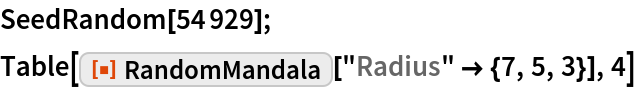Out=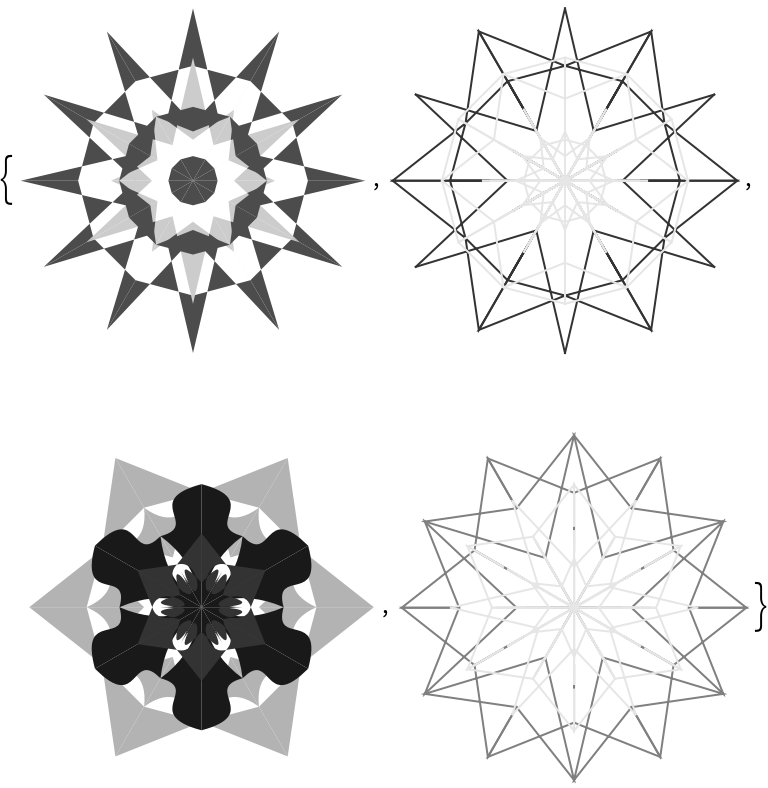In:=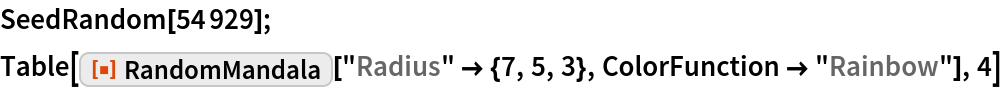Out=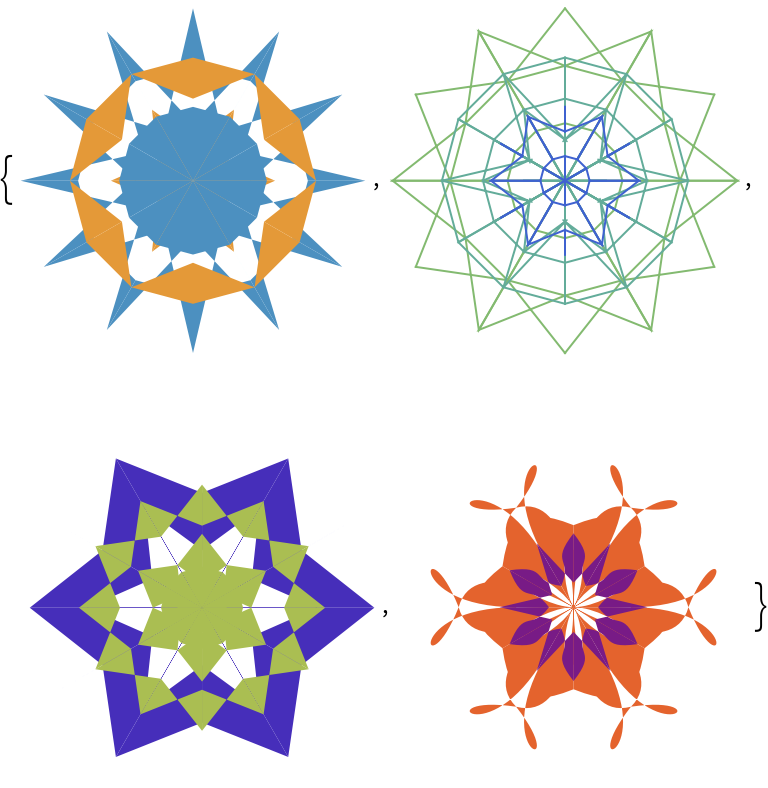### Options

#### ColorFunction

The function given to ColorFunction is run over Range[0,1,0.1] the obtained colors are used to colorize the mandala. By default no colorizing is done, .

ColorFunction can also take strings that are color schemes known by ColorData.

Here is a list of colorized mandalas using single-mandala mode:

 In:=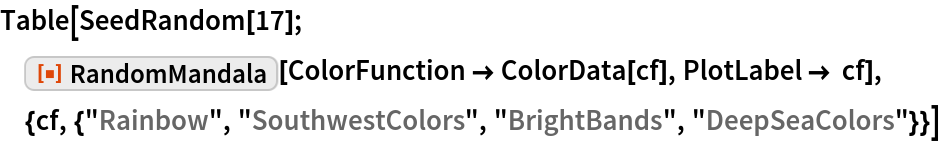Out=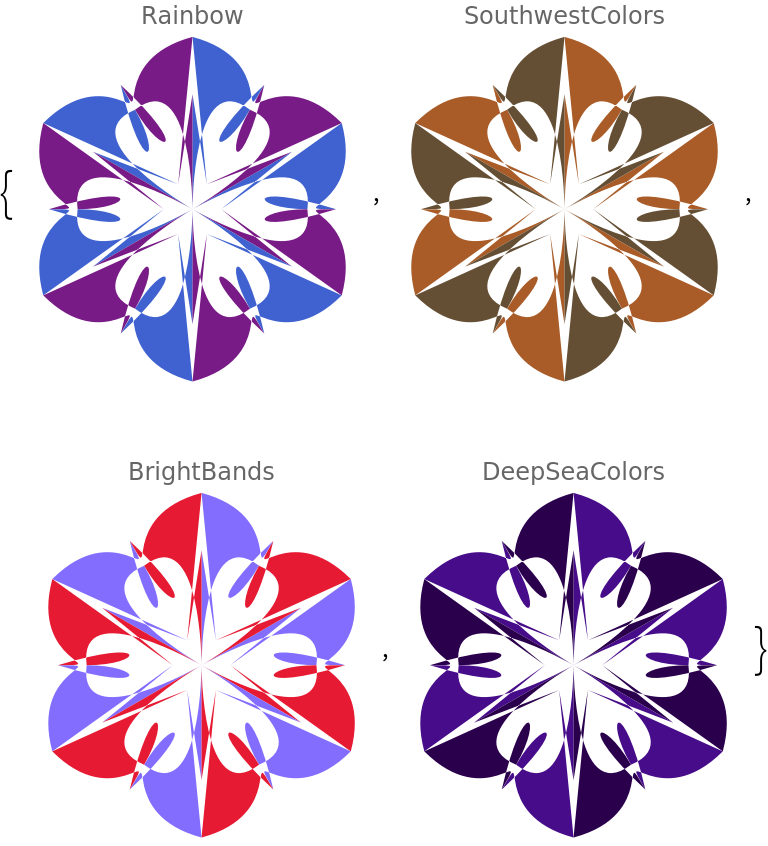Here are mandalas generated using multi-mandala mode:

 In:=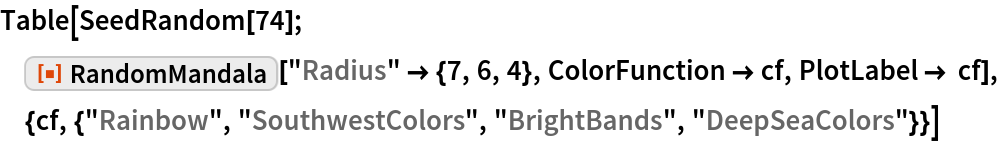Out=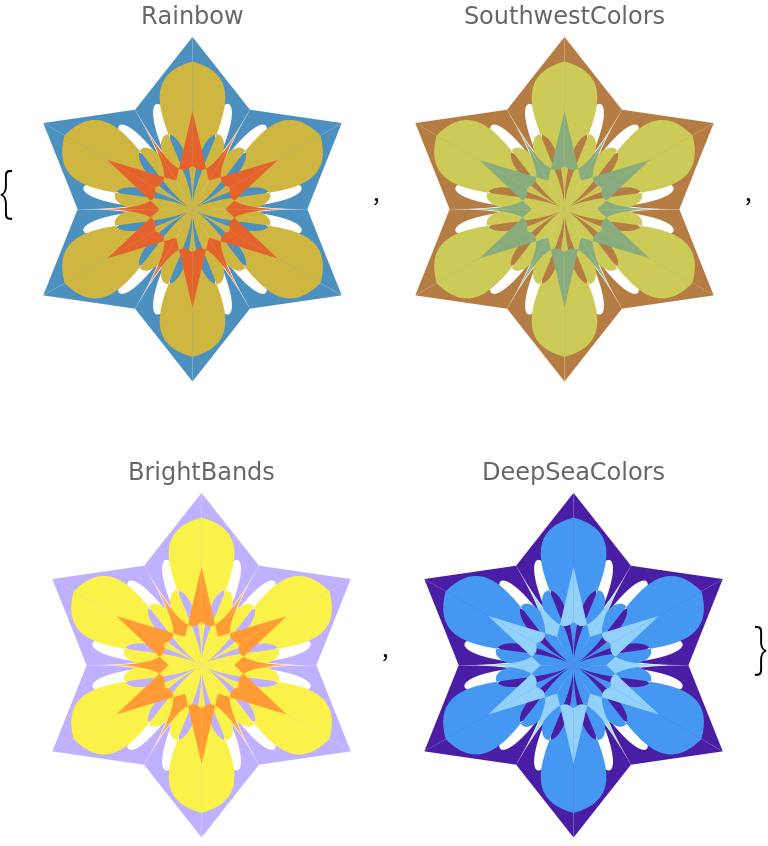#### ConnectingFunction

The option "ConnectingFunction" specifies which graphics primitive to be used over the seed segment points:

 In:=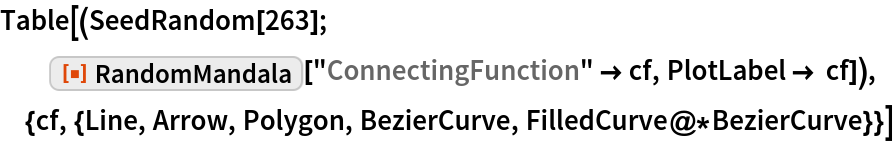Out=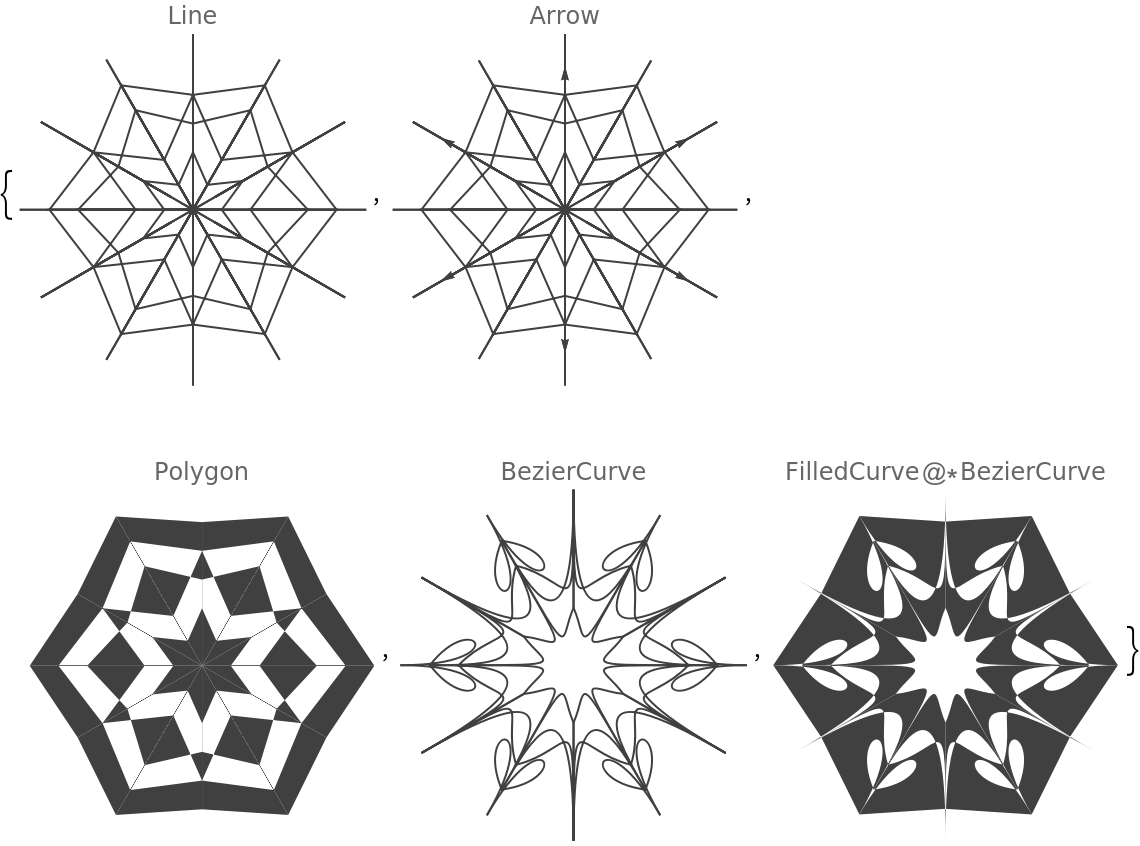#### KeepGridPoints

The option "KeepGridPoints" specifies should the points used to generate the seed segment be kept or not:

 In:=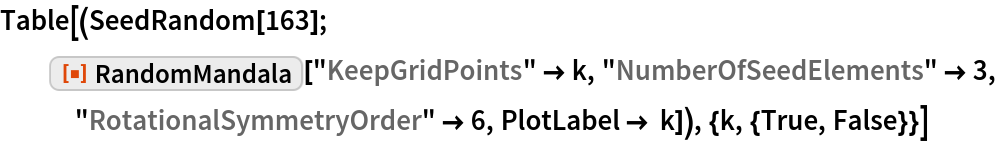Out=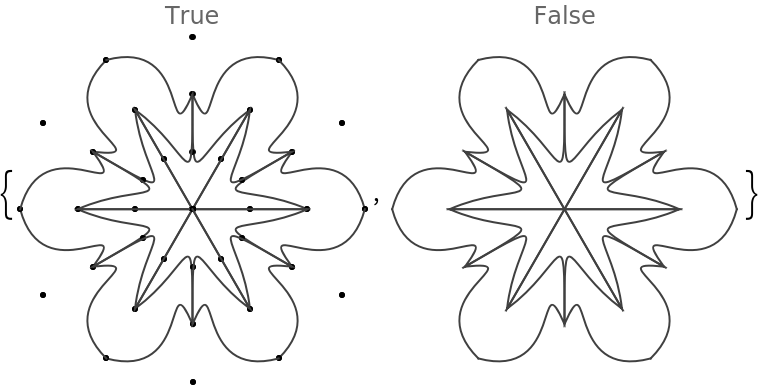In:=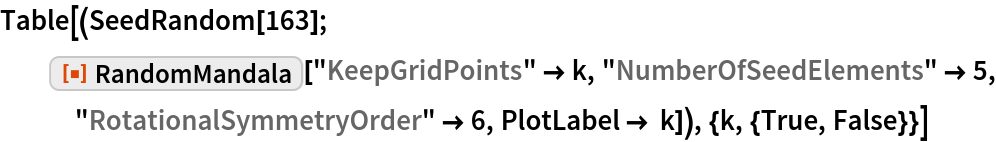Out=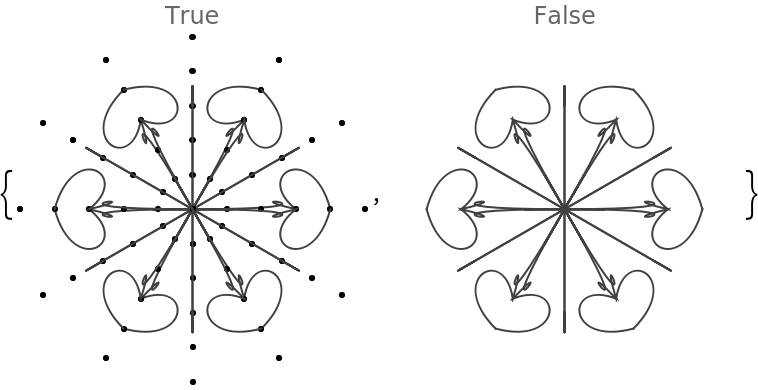#### NumberOfElements

The option "NumberOfElements" controls how may graphics elements are in the seed segment:

 In:=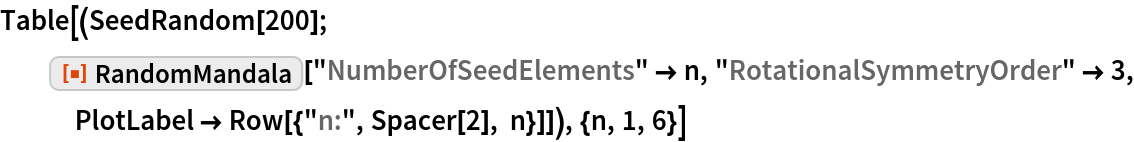Out=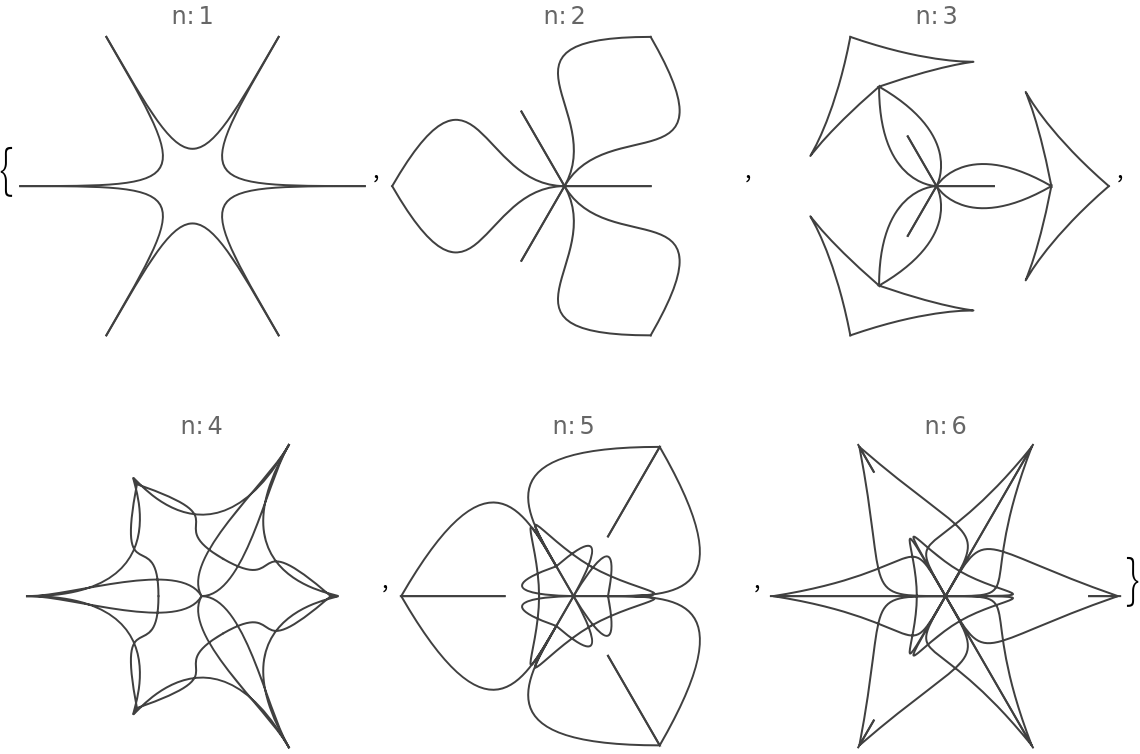In:=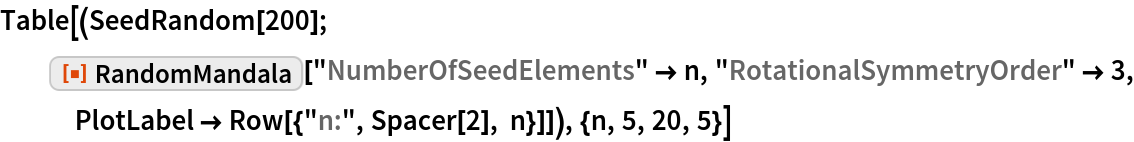Out=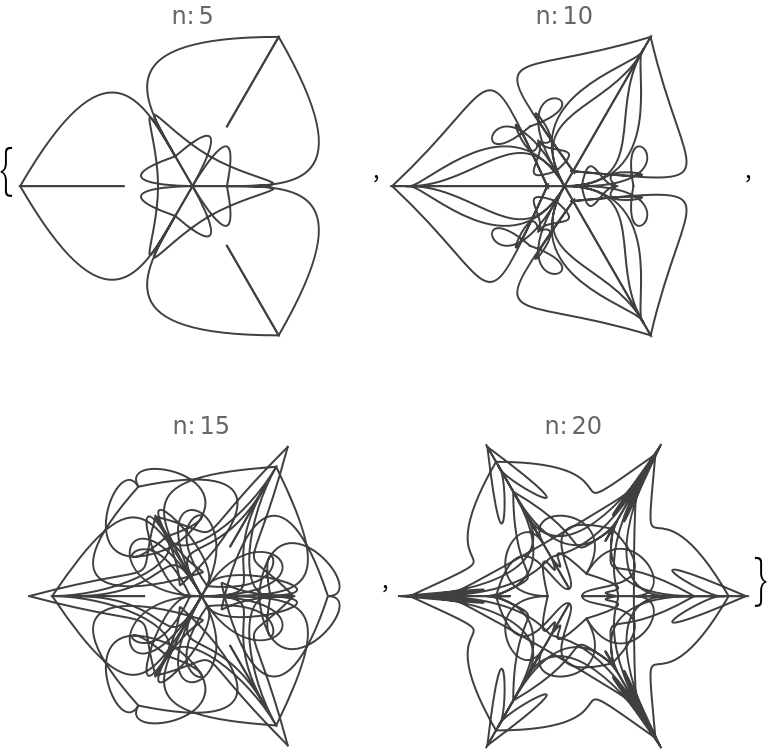If the value of “NumberOfElements” is Automatic in single-mandala mode 6 elements are used, and in multi-mandala mode 3 elements are used (for each mandala.)

In single-mandala mode the option "Radius" specifies the radius of the seed segment and the mandala:

 In:=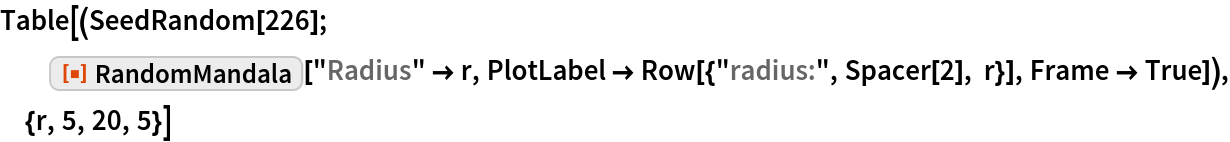Out=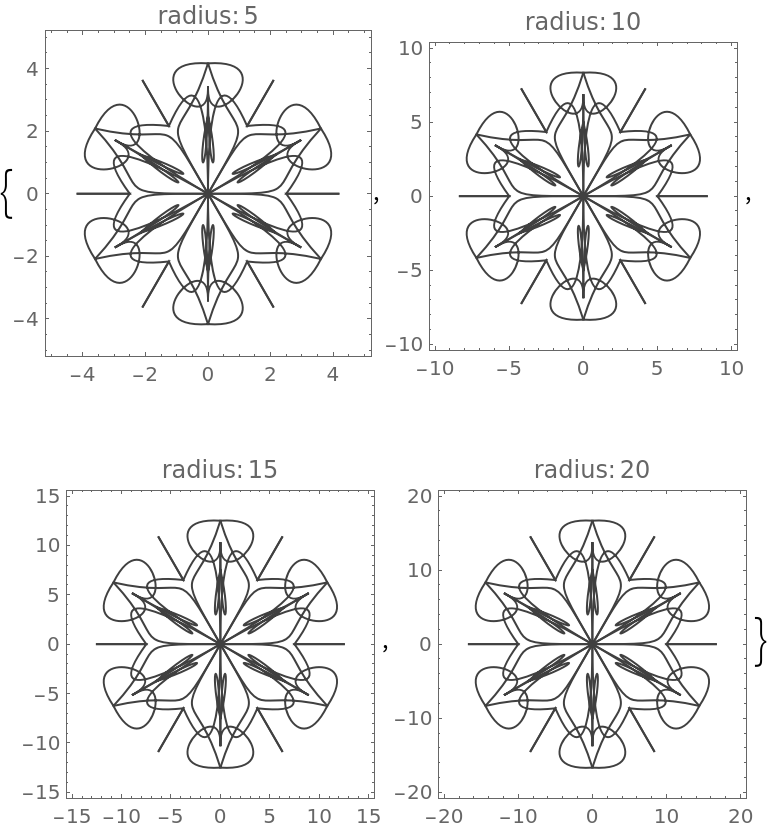If the value given to “Radius” is a list of positive numbers then multi-mandala mode is used.

If "Radius"{r1,,rk}, then for each ri is made a mandala with radius ri and the mandalas are drawn upon each other according to their radii order:

 In:=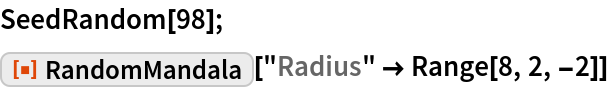Out=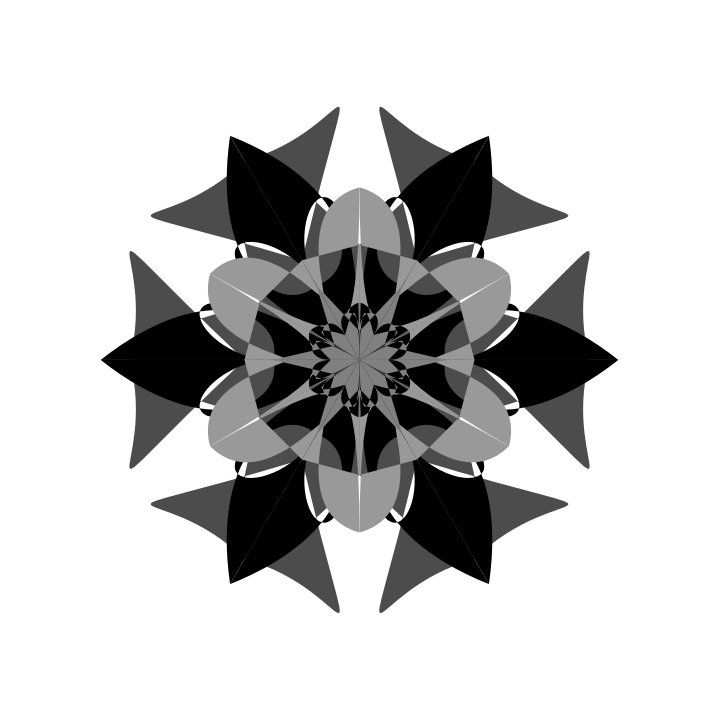Using the option ColorFunction colorized mandalas are obtained:

 In:=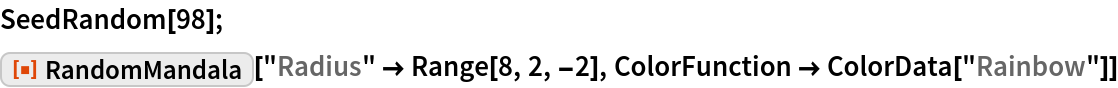Out=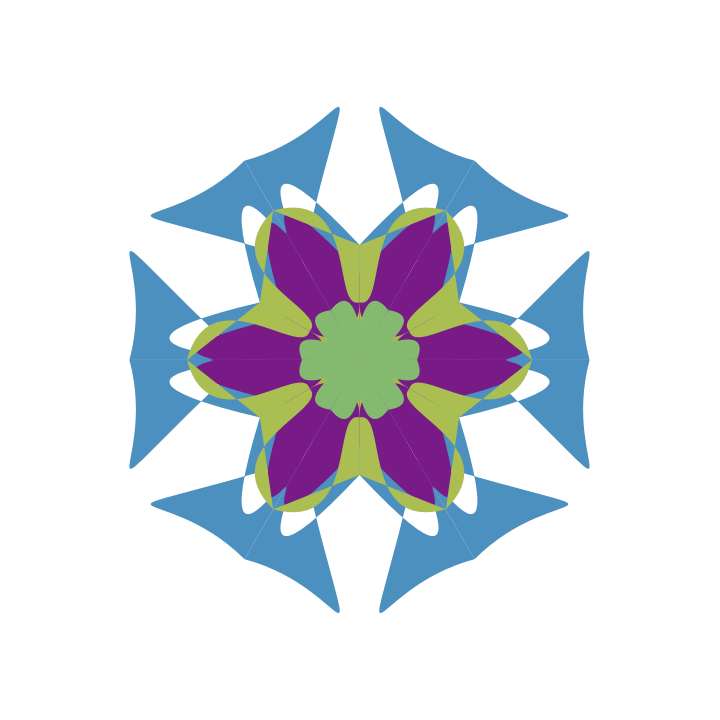#### RotationalSymmetryOrder

The "RotationalSymmetryOrder" option specifies how many copies of the seed segment comprise the mandala:

 In:=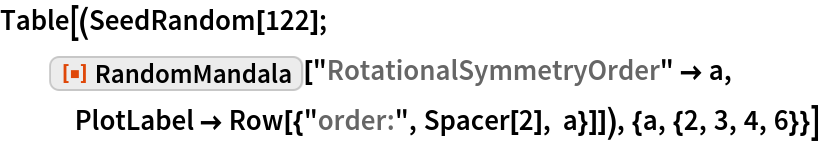Out=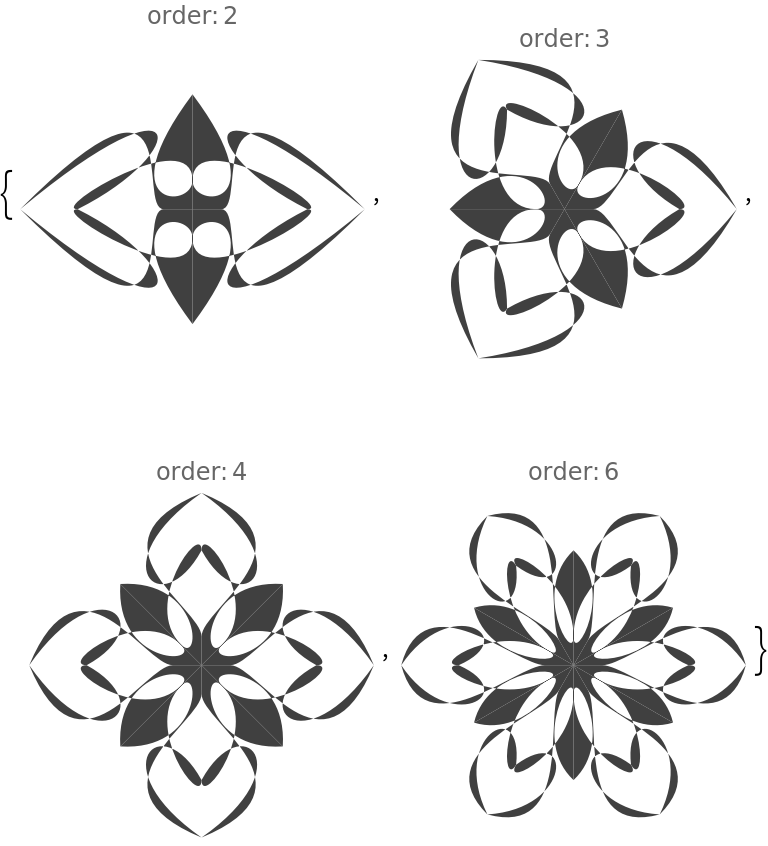In:=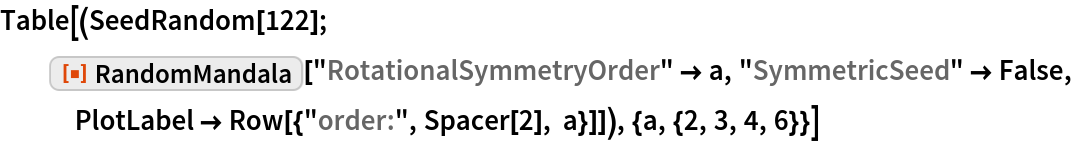Out=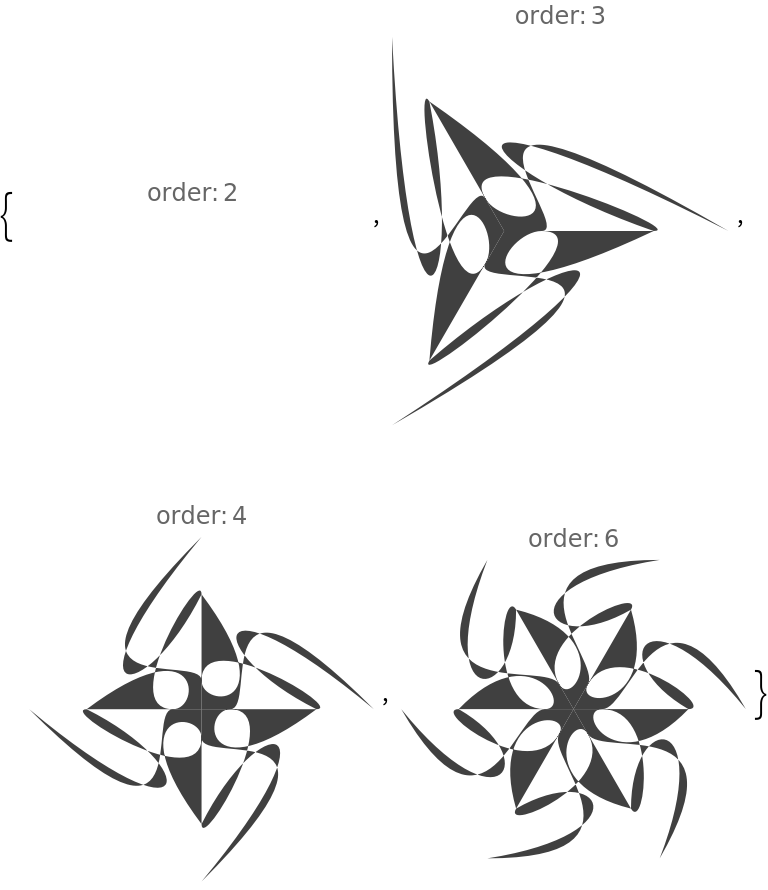#### SymmetricSeed

The option "SymmetricSeed" specifies should the seed segment be symmetric or not:

 In:=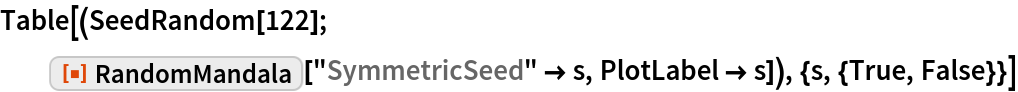Out=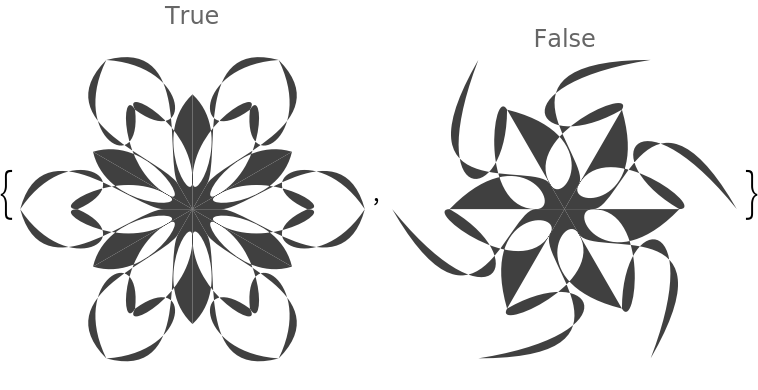### Possible Issues

In general, the rotational symmetry order should be an integer, but for didactic purposes it is permitted to be a positive real. If the specified rotational symmetry order is not an integer, then the produced mandalas will appear “open”:

 In:=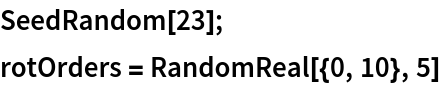Out=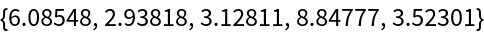In:=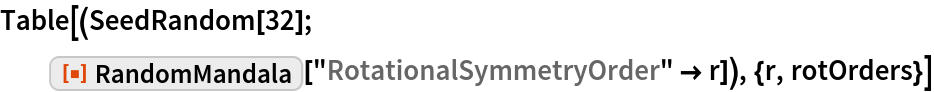Out=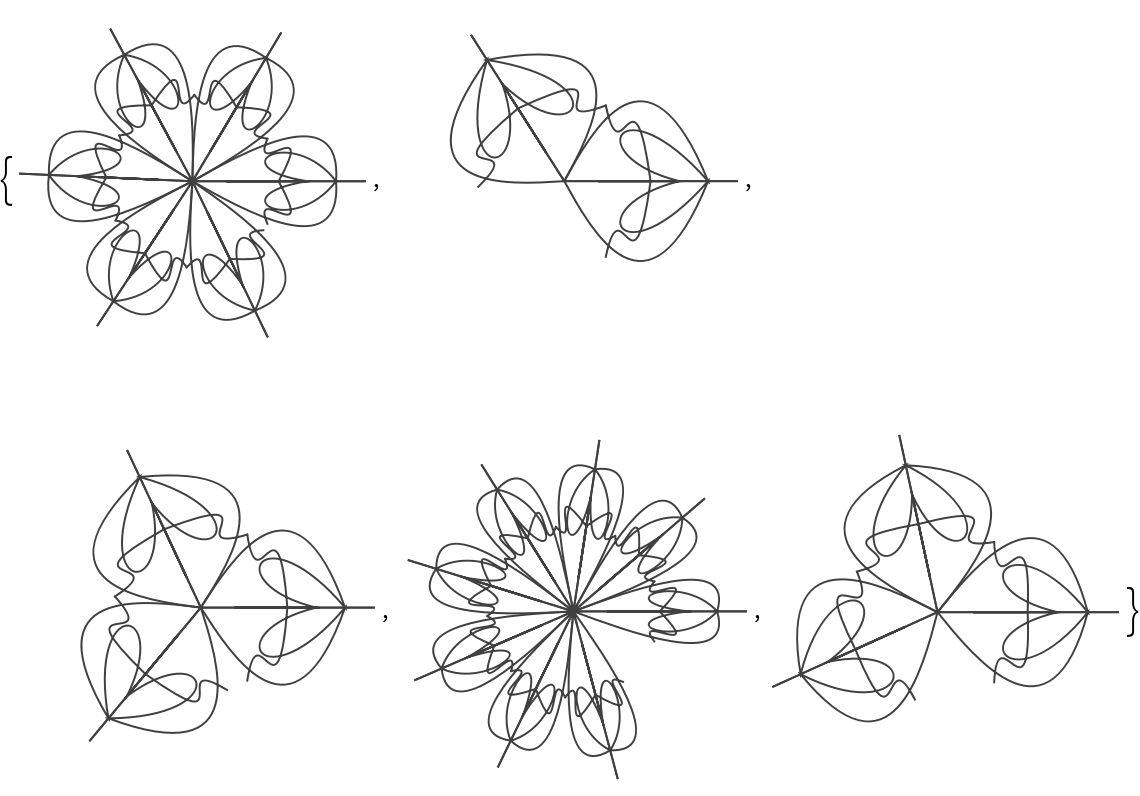In:=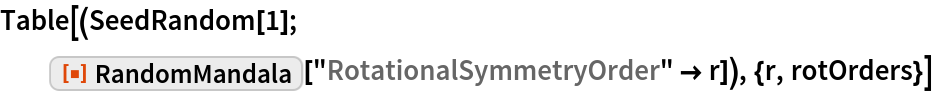Out=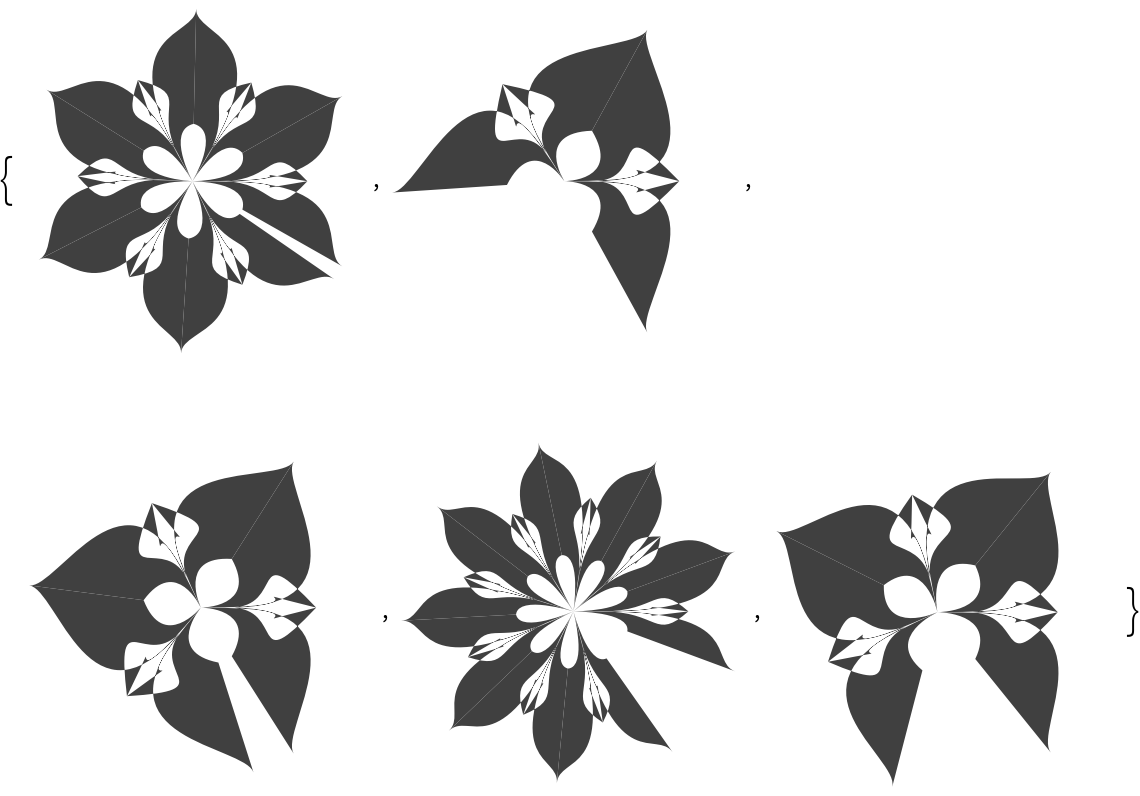### Neat Examples

#### A table of random mandalas

 In:=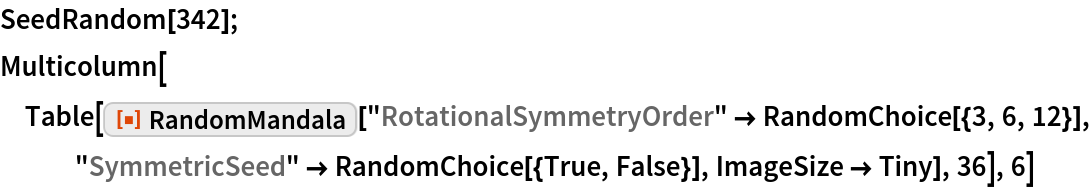Out=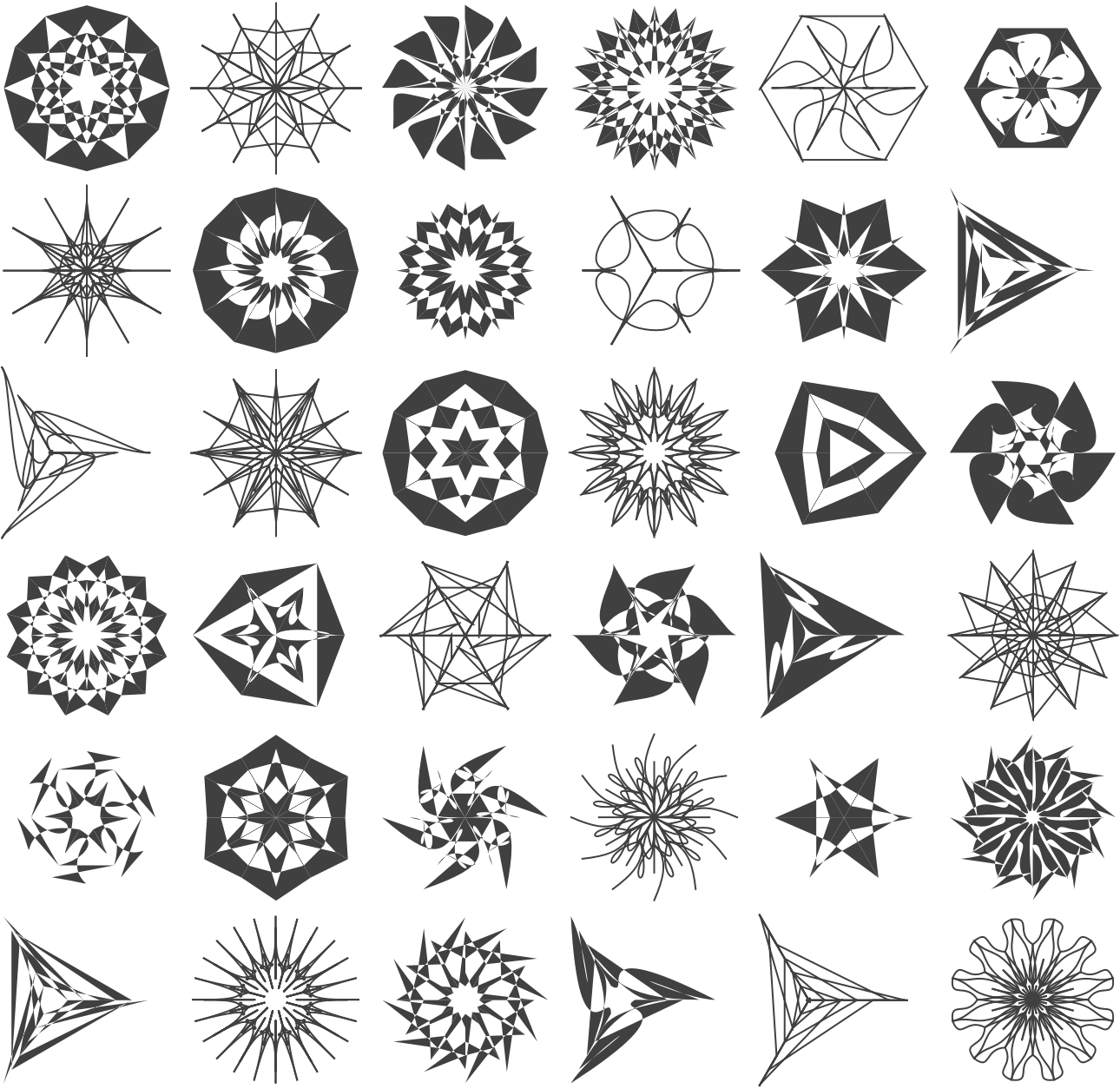#### A table of random colorized mandalas

 In:=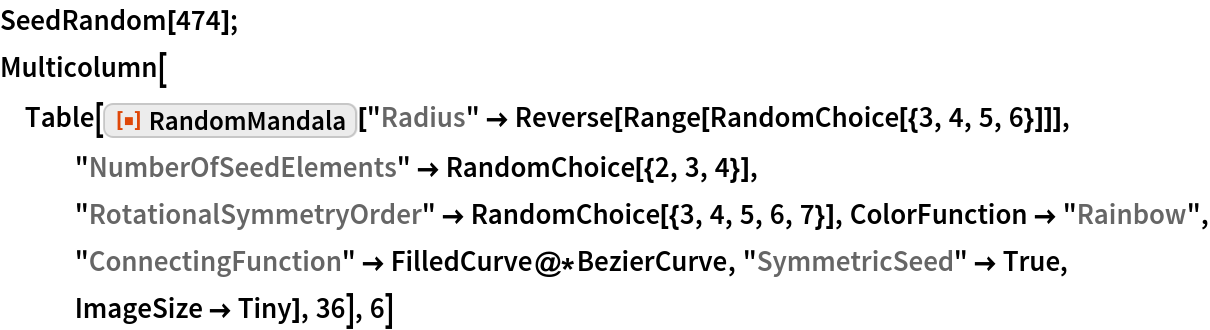Out=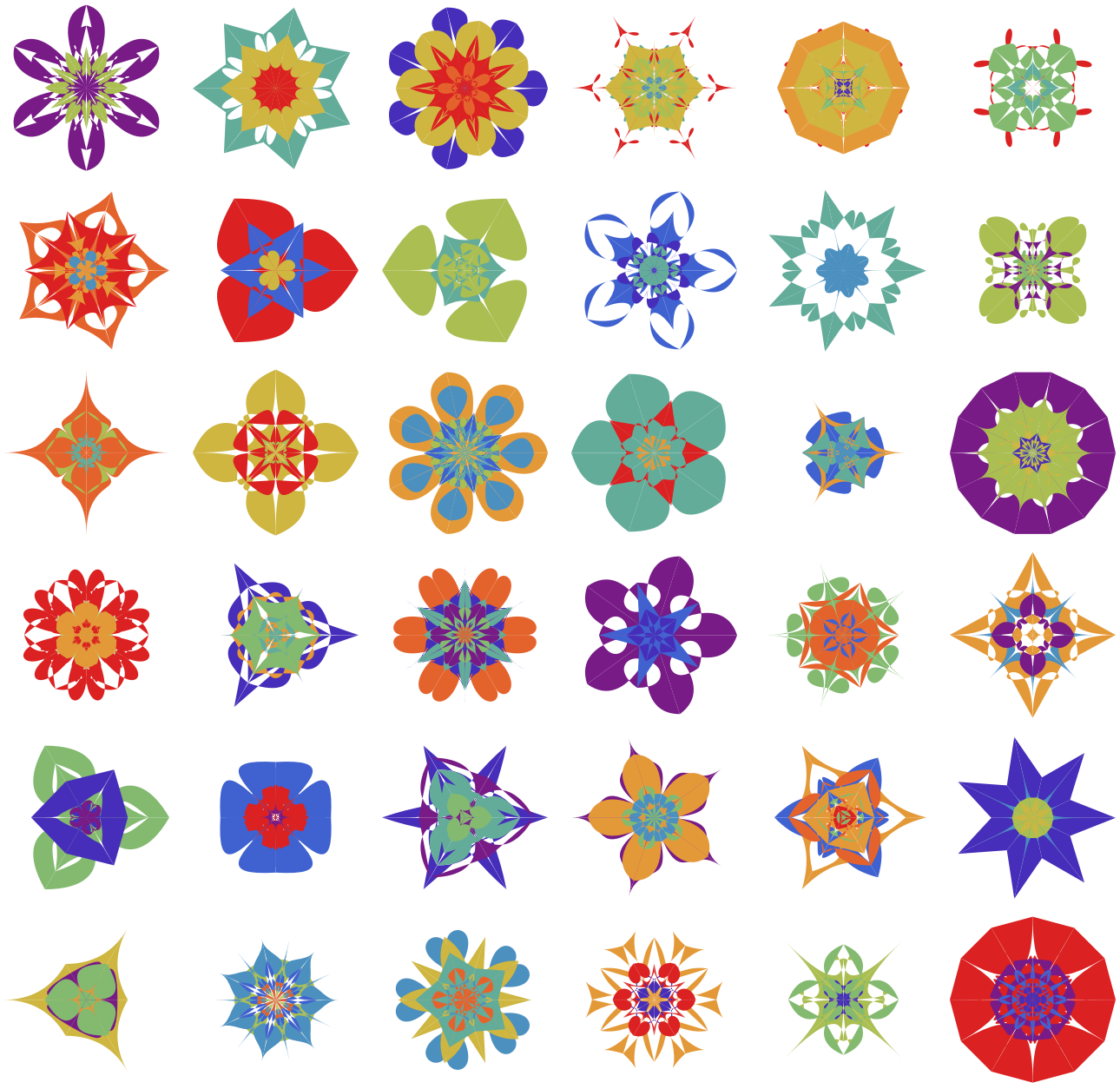#### A table of “open” colorized mandalas

 In:=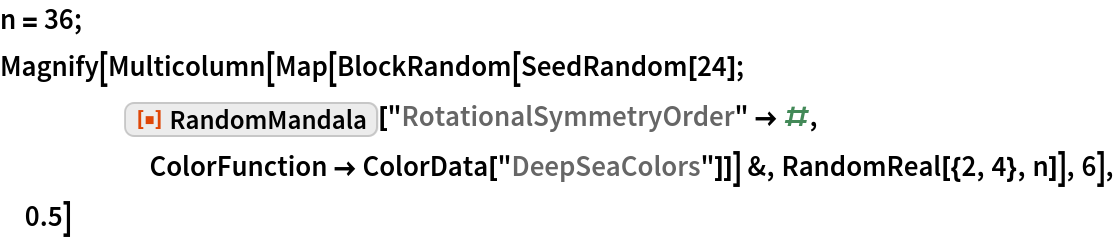Out=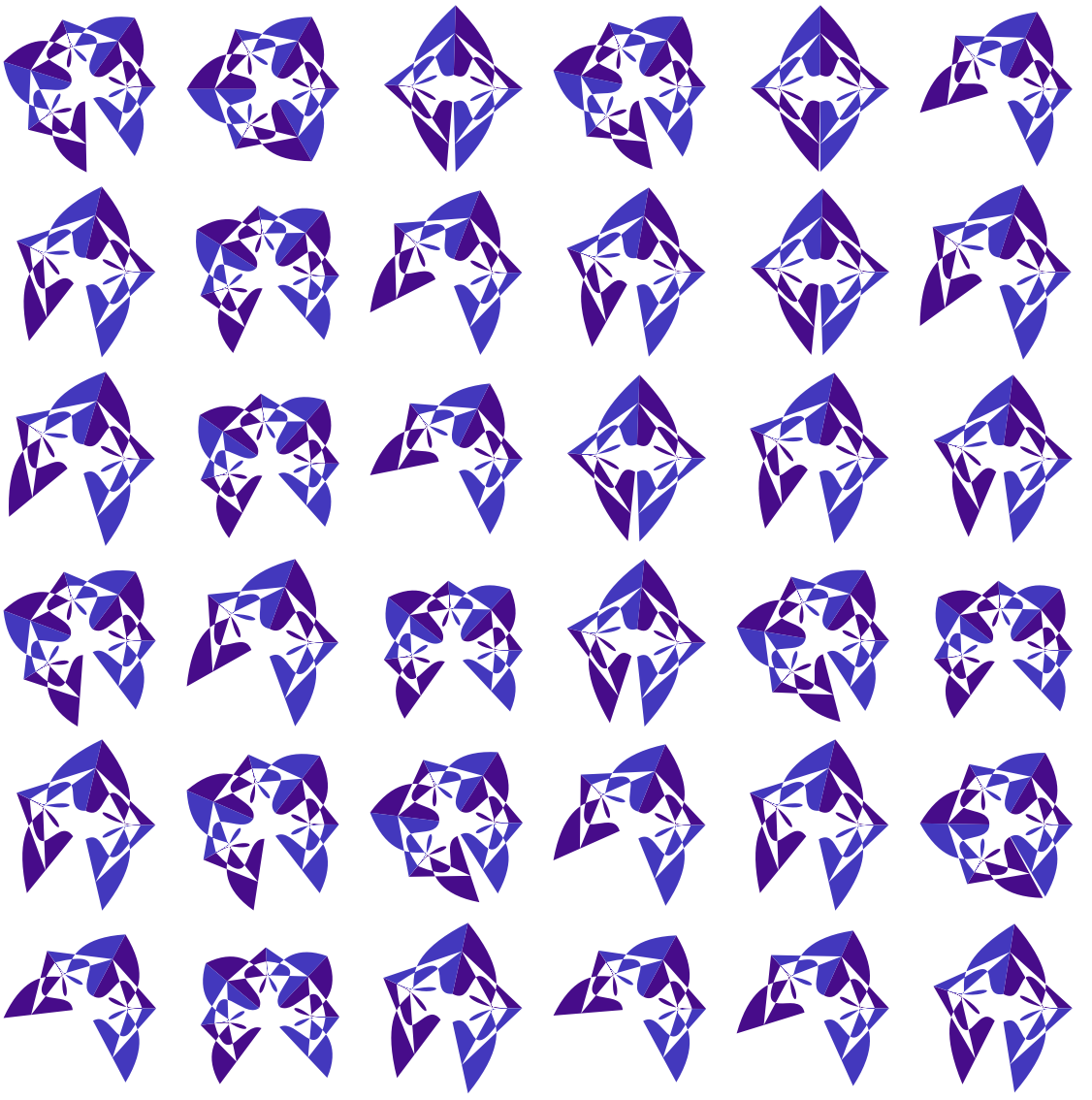#### Colored mandala images by blending

Make a list of random mandalas:

 In:=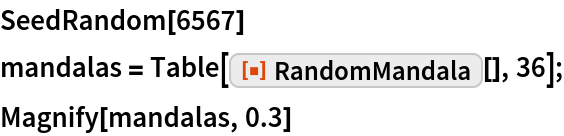Out=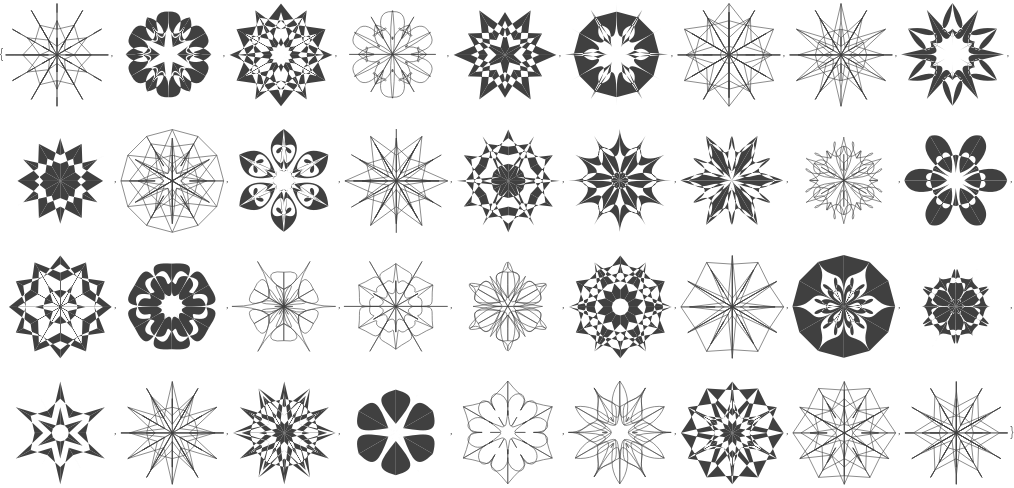Make images of the generated mandala graphics:

 In:=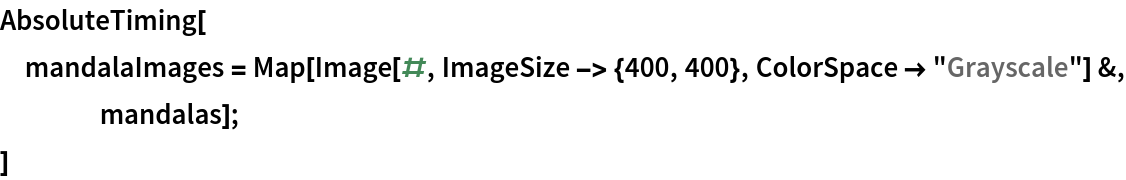Out=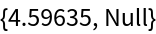Pick mandala images at random and blend them using a set of coloring schemes:

 In:=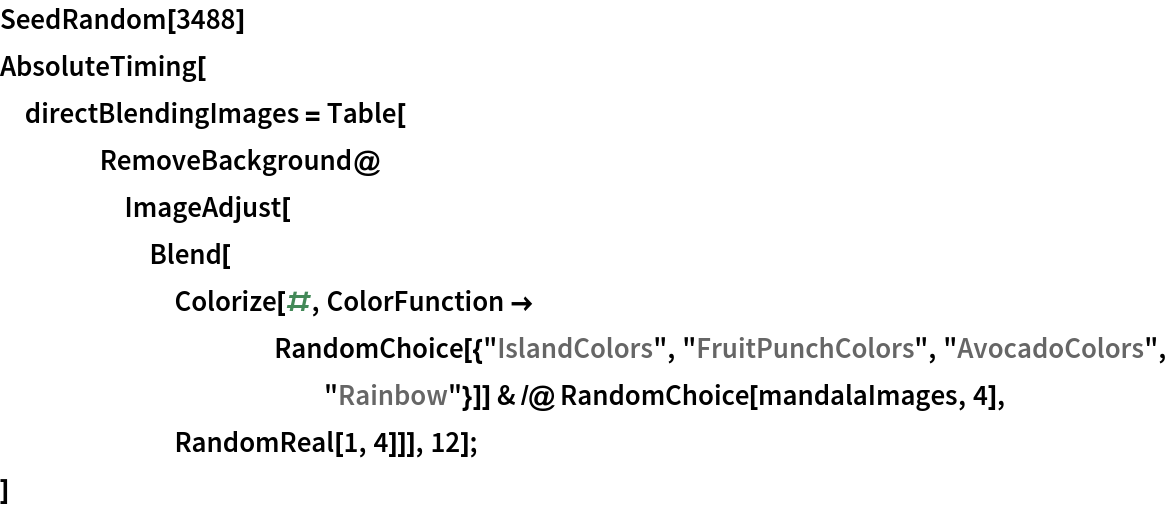Out=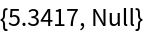Make a collage:

 In:=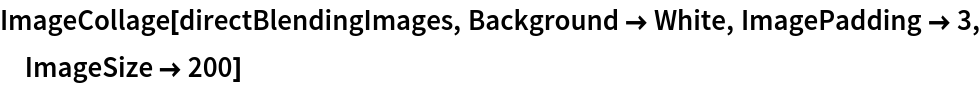Out=